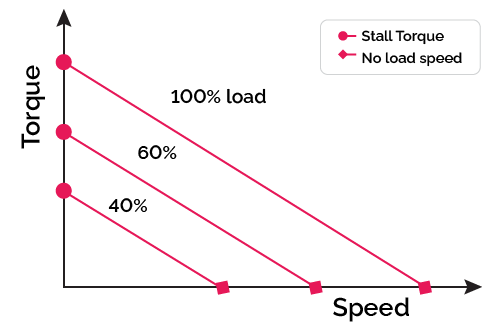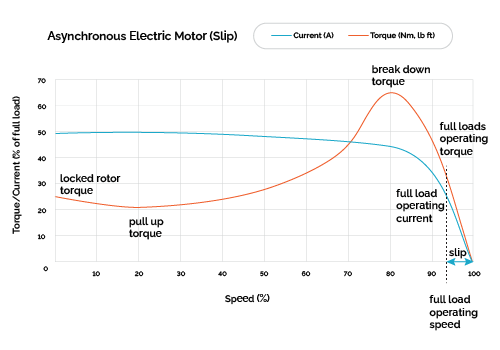# Electric Motor Torque Calculator

Created by Arturo Barrantes
Reviewed by Steven Wooding
Based on research by
Austin Hughes Electric Motors and Drives, 3rd edition Newnes (2006)
Last updated: Dec 28, 2022

The amazing electric motor torque calculator indicates the relationship between motor speed, power, and torque. Are you looking to determine how much torque you can get at a specific rotational speed? Then keep reading. We will discuss how an electric motor works and indicate formulas to calculate its torque. We also define, exclusively for alternate current, what is electrical motor slip.

💡 You might also like to check out our torque calculator and the torque to hp calculator after using this calculator.

## How does an electric motor work?

It is easy to determine whether the electric motor is working correctly, but few chosen know how they work. Keep reading; you are about to become one of the selected ones.

In case you think you have not seen an electric motor, you have one in the water pump of your house or your living room fan. Electric motors are everywhere, converting electrical energy into mechanical energy, making a shaft rotate. We have two main types of electrical motors:

• AC electrical motor: These work with alternating current. Alternating current has the characteristic of periodically reversing current direction and magnitude over time. Such a feature makes it perfect for creating alternating magnetic fields that rotate the motor.

• DC electrical motor: This type works with direct current. We call the armature its rotatory part and the stator its stationary part. The stator contains magnets that build a magnetic field that covers the armature. On the other side, we have the armature, which is energized by the DC power. The electrical flow through the armature creates an electromagnetic field. The interaction between the electromagnetic field and the stator magnetic field makes the armature rotate.

If you are wondering which cable size to use for your new electrical motor, you can check our set of wire size calculators. We have a specific set of tools for DC and AC motors.

## What is the electric motor torque?

In both cases described above, the shaft rotates with a certain angular speed producing torque. We can use such torque to rotate driven equipment like impellers in water pumps. In other words, electric motor torque is the amount of rotational force the motor develops.

In both types of electrical motors, torque depends on the intensity of the magnetic fluxes inside the motor; however, their speed and torque relation are different. In direct current, a high-torque electric motor will rotate considerably slower than at other loads. You can see this in the figure below:That is why the phrase: "high torque low-speed electric motor" is mostly related to DC motors. On the other hand, AC electric motor experiences its maximum torque at a specific rotating speed. Engineers call such torque breakdown torque. Check it in the next picture:## What is slip in electrical motors?

In the above picture, note the "slip" text in the x-axis next to 100% speed. Slip is a value that only applies to alternate current motors. It represents the difference between synchronous velocity (the rotating speed of the magnetic field inside the motor) and the shaft speed. Our powerful electric motor torque calculator can also calculate it. Here you can see its formula:

$\small s=\rm\frac{rpm_{noload}-rpm_{load}}{rpm_{noload}} \times 100\%$

Slip is an important value since it indicates how much less speed you get running the electric motor compared to its synchronous velocity. From the graph, you can notice that maximum torque (breakdown torque) appears at a specific slip value which is data that manufacturers provide. See the catalog of a famous .

## How to calculate the electric motor torque?

Independently of the current source, there is one main formula that relates shaft revolutions per minute, torque, and power:

$\small T= 60 \times P/(2 \times \pi \times\rm rpm),$

where:

• $T$Torque: also referred to as electric motor torque, represents the amount of rotational force that the motor develops;
• $P$Power: indicates the output power that can be used from the shaft; and
• $\rm rpm$Motor speed: Measures the rotation speed of the shaft.

In AC electric motors, we have two rotation speeds: synchronous speed or magnetic field speed and real rotation speed:

• $\rm rpm_{synch}$ — Synchronous revolutions per minute: Indicates the rotational speed of the magnetic field. It is controlled by the voltage frequency and the number of electric motor poles.
• $\rm rpm_{load}$ — Motor speed with load: Represents the real rotation speed when the motor is under load. It is less than the synchronous speed.

Synchronous revolutions per minute can be calculated as follows:

$\small {\rm rpm_{synch}} = \frac{120 \times f} {p},$

where:

• $f$ — Supply frequency: indicates the supply voltage frequency; and
• $p$ — Number of poles: Represents the number of permanent magnet poles for the rotating rotor. A paired set of magnets with north and south represents two poles.

The electric motor torque calculator includes a feature where, by adding the maximum slip, you can get the operating rotating speed or vice versa. In other words, if you add the motor operating speed, you obtain the slip. Such a feature helps determine whether the motor is operating at its maximum torque or not.

## FAQ

### How much torque is produced by a 1 HP motor at 60Hz?

3.96 N·m, in case we are talking about an 1800 rpm motor, or 1.98 N·m for 3600 rpm. The Omni Calculator tool electric motor torque calculator can provide the torque for different rotating speeds, or you can do as follows:

1. For the 1800 rpm case, apply the power, torque, and velocity relation:

Power = 2 × 𝜋 × rpm × T / 60

Solve for T. Use Power = 1 hp and rpm = 1800 rpm.

2. We get torque (T) = 3.96 N·m.

### What is the torque of 1 kW, 4 pole motor?

5.3 N·m. We can use Omni Calculator tool electric motor torque calculator or do as follows:

1. Find out the motor speed. Angular velocity (w), in rpm = 120 × frequency / Number of poles. Assuming 60 Hz, we get 1800 rpm.

2. Apply the power, torque, and velocity relation:

Power = 2 × 𝜋 × rpm × T / 60

Solve for T. Use Power = 1000 watts.

3. We get Torque (T) = 5.3 N·m.

### What is breakdown torque in AC electrical motors?

Breakdown torque is the maximum torque an AC motor can reach. In electric motors, when they are starting, torque increases while the speed increases until they get to a maximum torque point. Afterward, if the motor can further increase its speed, we see how drastically the torque decreases to zero when it reaches full speed. Remember that an electric motor will never reach its synchronous velocity.

### How to build a high torque electrical motor torque output?

For increasing electric motor torque from a design point of view, you need more poles inside the motor. Poles refer to the set of paired magnets that create the magnetic field required to rotate the shaft. The more poles you have inside an electric motor, the lower the speed, and as a consequence, the higher the torque. Engineers call this group: high torque, low-speed electric motors.

Arturo Barrantes
Power and torque
Supply frequency
Hz
Number of poles
Motor speed
rpm
Power
W
Torque
N·m
Electric motor slip (only for AC motors)
rpm
Slip
%
People also viewed…

### Books vs e-Books